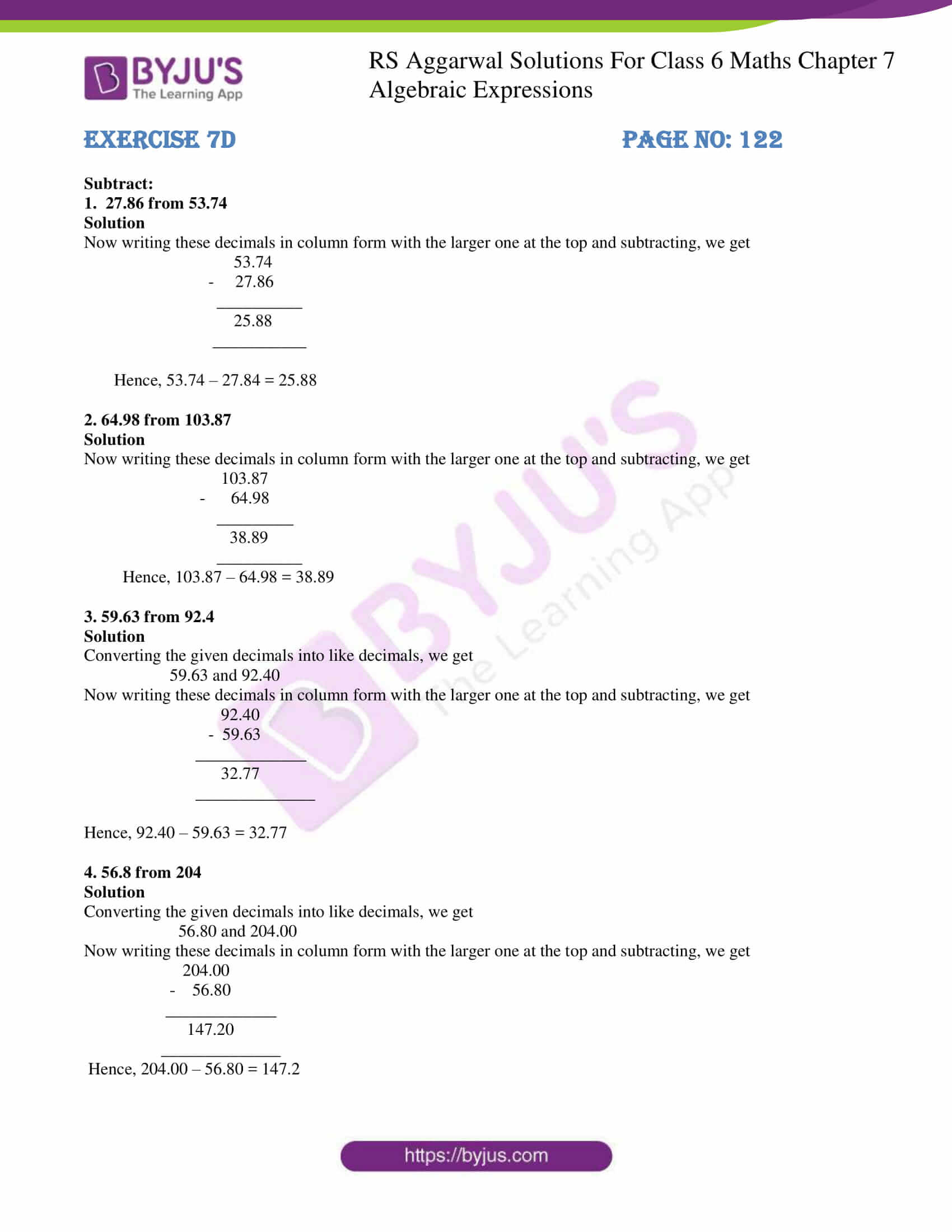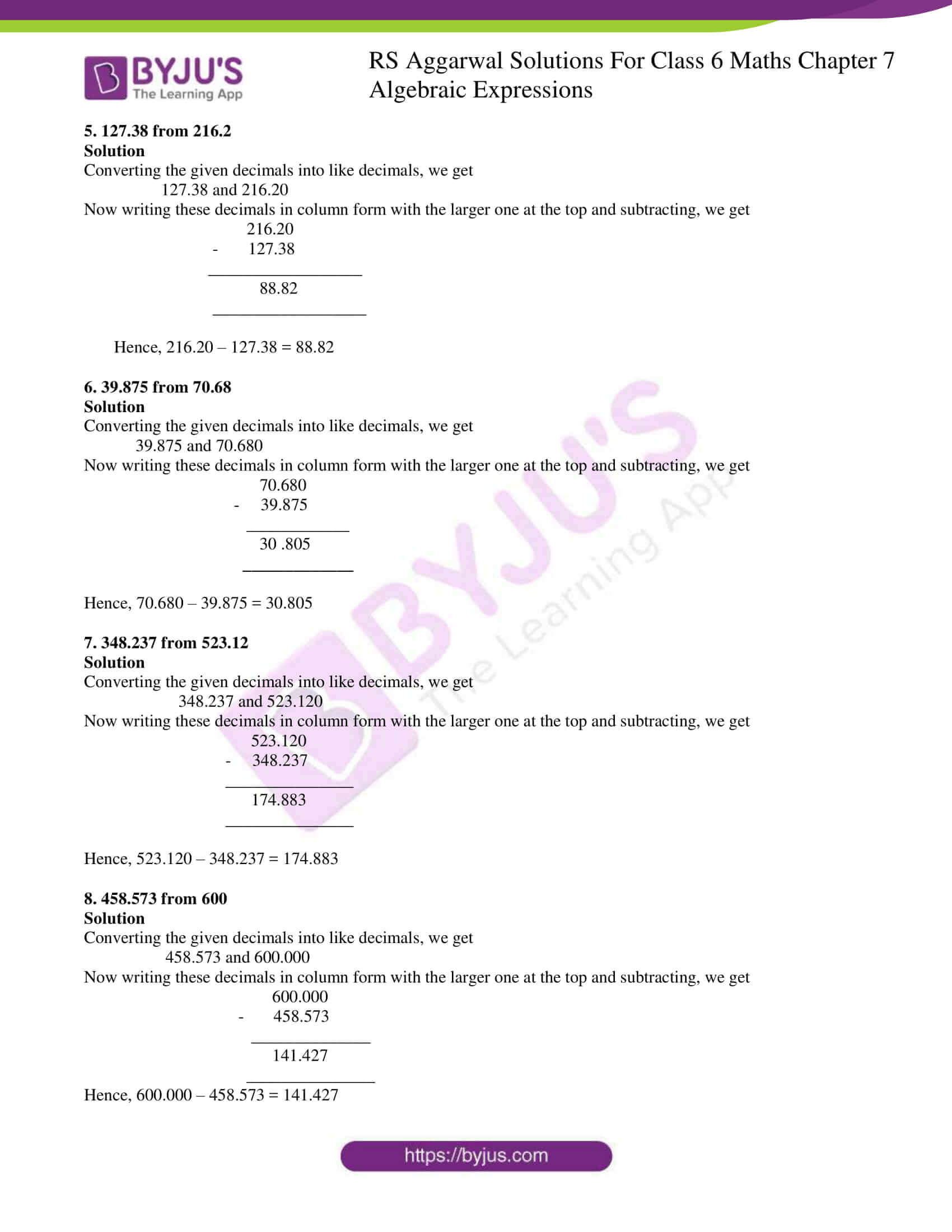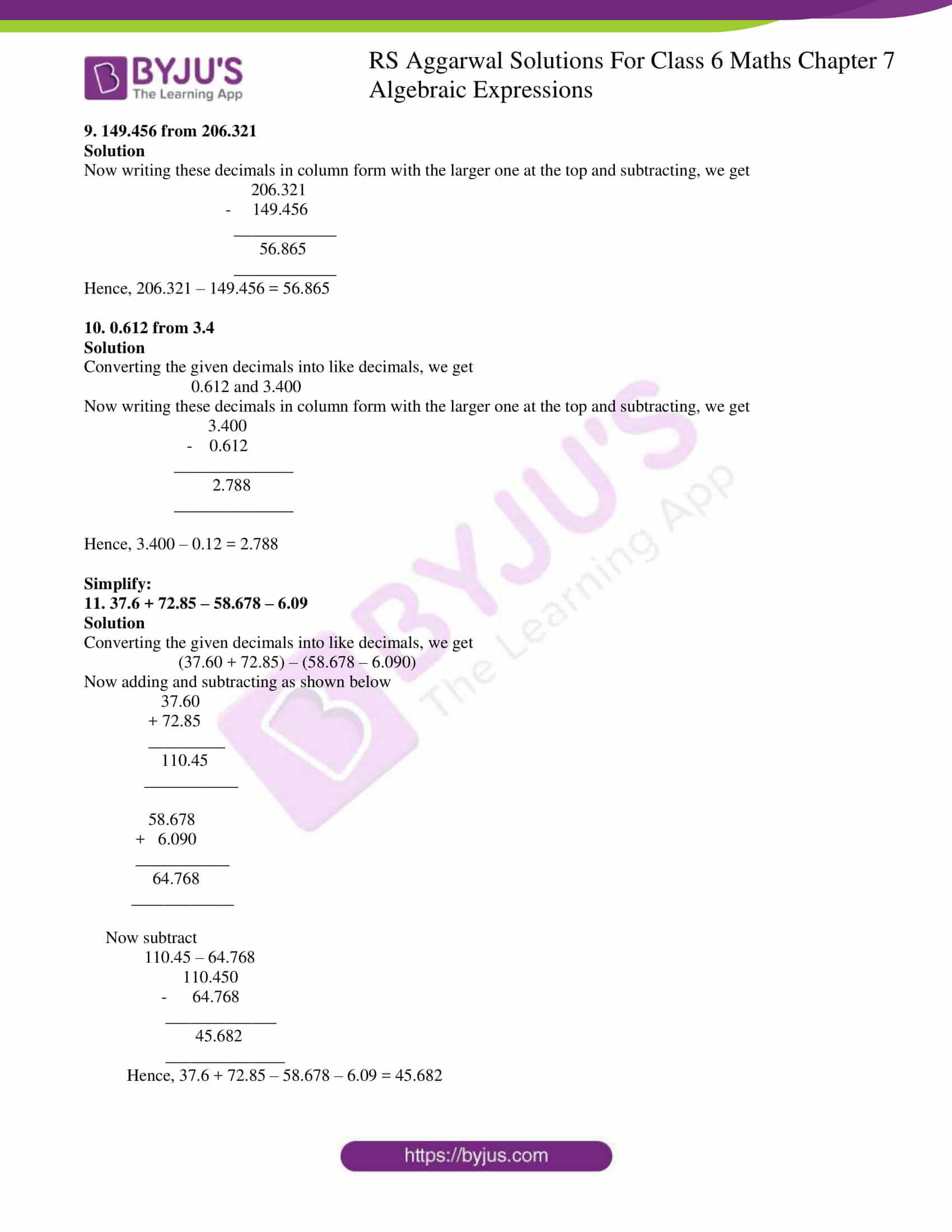# RS Aggarwal Solutions for Class 6 Chapter 7 Decimals Exercise 7D

RS Aggarwal Solutions for Class 6 Chapter 7 Decimals Exercise 7D are here in PDF format. This exercise has the questions related to subtraction of Decimals along with examples to solve the problems faster. By practicing the problems, students achieve an idea about the problems that would appear on the subtraction of Decimals. Faculty at BYJU’S have solved the problems for the students as per the latest syllabus.

## Download PDF of RS Aggarwal Solutions for Class 6 Chapter 7 Decimals Exercise 7D### Access answers to Maths RS Aggarwal Solutions for Class 6 Chapter 7 Decimals Exercise 7D

Subtract:

1. 27.86 from 53.74

Solution

Now writing these decimals in column form with the larger one at the top and subtracting, we get

53.74

– 27.86

__________

25.88

___________

Hence, 53.74 – 27.84 = 25.88

2. 64.98 from 103.87

Solution

Now writing these decimals in column form with the larger one at the top and subtracting, we get

103.87

– 64.98

_________

38.89

__________

Hence, 103.87 – 64.98 = 38.89

3. 59.63 from 92.4

Solution

Converting the given decimals into like decimals, we get

59.63 and 92.40

Now writing these decimals in column form with the larger one at the top and subtracting, we get

92.40

– 59.63

_____________

32.77

______________

Hence, 92.40 – 59.63 = 32.77

4. 56.8 from 204

Solution

Converting the given decimals into like decimals, we get

56.80 and 204.00

Now writing these decimals in column form with the larger one at the top and subtracting, we get

204.00

– 56.80

_____________

147.20

______________

Hence, 204.00 – 56.80 = 147.2

5. 127.38 from 216.2

Solution

Converting the given decimals into like decimals, we get

127.38 and 216.20

Now writing these decimals in column form with the larger one at the top and subtracting, we get

216.20

– 127.38

__________________

88.82

__________________

Hence, 216.20 – 127.38 = 88.82

6. 39.875 from 70.68

Solution

Converting the given decimals into like decimals, we get

39.875 and 70.680

Now writing these decimals in column form with the larger one at the top and subtracting, we get

70.680

– 39.875

____________

30 .805

_____________

Hence, 70.680 – 39.875 = 30.805

7. 348.237 from 523.12

Solution

Converting the given decimals into like decimals, we get

348.237 and 523.120

Now writing these decimals in column form with the larger one at the top and subtracting, we get

523.120

– 348.237

_______________

174.883

_______________

Hence, 523.120 – 348.237 = 174.883

8. 458.573 from 600

Solution

Converting the given decimals into like decimals, we get

458.573 and 600.000

Now writing these decimals in column form with the larger one at the top and subtracting, we get

600.000

– 458.573

______________

141.427

_______________

Hence, 600.000 – 458.573 = 141.427

9. 149.456 from 206.321

Solution

Now writing these decimals in column form with the larger one at the top and subtracting, we get

206.321

– 149.456

____________

56.865

____________

Hence, 206.321 – 149.456 = 56.865

10. 0.612 from 3.4

Solution

Converting the given decimals into like decimals, we get

0.612 and 3.400

Now writing these decimals in column form with the larger one at the top and subtracting, we get

3.400

– 0.612

______________

2.788

______________

Hence, 3.400 – 0.12 = 2.788

Simplify:

11. 37.6 + 72.85 – 58.678 – 6.09

Solution

Converting the given decimals into like decimals, we get

(37.60 + 72.85) – (58.678 – 6.090)

Now adding and subtracting as shown below

37.60

+ 72.85

_________

110.45

___________

58.678

+ 6.090

___________

64.768

____________

Now subtract

110.45 – 64.768

110.450

– 64.768

_____________

45.682

______________

Hence, 37.6 + 72.85 – 58.678 – 6.09 = 45.682